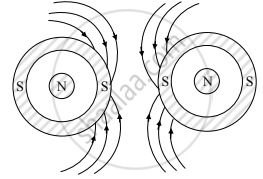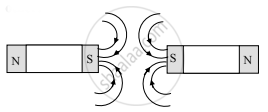# Answer the following question in detail. Draw a diagram to illustrate the magnetic lines of force between the south poles of two such magnets. - Physics

Diagram

Answer the following question in detail.

Draw a diagram to illustrate the magnetic lines of force between the south poles of two such magnets.

#### Solution

• For south poles of such circular magnets facing each other:• For south poles of bar magnets facing each other:Concept: Magnetic Lines of Force and Magnetic Field
Is there an error in this question or solution?

#### APPEARS IN

Balbharati Physics 11th Standard Maharashtra State Board
Chapter 12 Magnetism
Exercises | Q 4. (iv) | Page 228